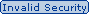#### Which of the following correctly pairs the dotted decimal subnet mask with the correct number of binary bits that represent the subnet mask?Send Via Email (Need Reachable Email ID)## 99.9% OFF All In One Supper Package

Which of the following correctly pairs the dotted decimal subnet mask with the correct number of binary bits that represent the subnet mask?
A. 255.255.255.192 and /25
B. 255.255.255.248 and /28
C. 255.255.255.224 and /26
D. 255.255.255.248 and /27
E. 255.255.255.240 and /28
F. 255.255.255.240 and /16